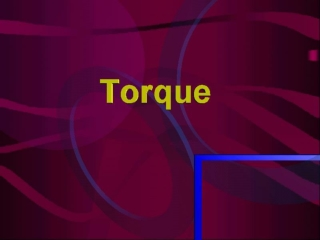DownloadDownload PresentationCausing Rotational Motion

Causing Rotational Motion

Download PresentationCausing Rotational Motion

- - - - - - - - - - - - - - - - - - - - - - - - - - - E N D - - - - - - - - - - - - - - - - - - - - - - - - - - -
Presentation Transcript

1. Causing Rotational Motion • In order to make an object start rotating about an axis, a force is required • However, not only the amount of force applied but also the position from the axis to which it is applied is important in determining the amount of rotation (angular acceleration • Distance from axis is called the Lever Arm

2. Torque Definition • Combination of amount of force and distance from axis is called Torque (τ) • A torque is necessary in order to provide angular acceleration • τ = rF r- dist from axis • Units - N m • In order to use this equation, the force must be perpendicular to the axis of rotation

3. Example 1 • A biceps muscle exerts a vertical force on the lower arm as shown. Calculate the torque acting on the lower arm if the muscle is attached 5.0 cm from the elbow.

4. Example 2 • A wrench is pulled by a force of 30 N at an angle of 30° with the axis and a distance of 15cm from its pivot end. What torque is acting on it?

5. Equilibrium • Occurs when total force acting balance and no rotational motion occurs • In order for equilibrium to exist, the total torque acting in the clockwise direction must balance the total torque acting in the counterclockwise direction

6. See-Saw • When stationary, the total τ clockwise is equal to the τ counterclockwise • If kid A pushes up with more force, the τ is no longer balanced (more force clockwise) and the see-saw begins to rotate clockwise with an angular velocity

7. Example 3 • Two people are sitting on a seesaw that is 2.5 m long. One has a mass of 40 kg and the other a mass of 60 kg. Where would the support on the see-saw need to be placed in order to produce equilibrium?

8. Example 4 • A 7.0 m beam is hanging from the ceiling by two ropes on its ends. The bar has a mass of 300 kg and it is non-uniform, with its center of mass 3.0 m from one end. A 400kg mass is hung from the beam’s light end and a 200 kg mass is hung from its heavy end. What force is acting on each rope?